# Chebyshev theorems on prime numbers

(diff) ← Older revision | Latest revision (diff) | Newer revision → (diff)

The theorems 1)–8) on the distribution of prime numbers, proved by P.L. Chebyshev  in 1848–1850.

Let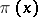be the number of primes not exceeding, letbe an integer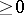, letbe a prime number, letbe the natural logarithm of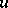, and let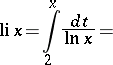(*)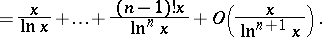1) For anythe sum of the serieshas a finite limit as.

2) For arbitrary small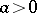and arbitrary large, the functionsatisfies the two inequalitiesinfinitely often.

3) The fractioncannot have a limit distinct from 1 as.

4) Ifcan be expressed algebraically in,and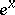up to order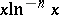, then the expression must be (*). After this, Chebyshev introduced two new distribution functions for prime numbers — the Chebyshev functions (cf. Chebyshev function)and actually determined the order of growth of these functions. Hence he was the first to obtain the order of growth ofand of the-th prime number. More precisely, he proved:

5) If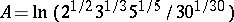, then for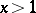the inequalities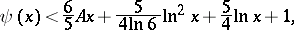hold.

6) Forlarger than some, the inequality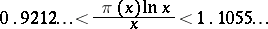holds.

7) There exist constants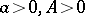such that for all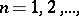the-th prime numbersatisfies the inequalities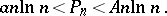8) Forthere is at least one prime number in the interval(Bertrand's postulate).

The main idea of the method of proof of 1)–4) lies in the study of the behaviour of the quantities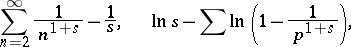and their derivatives as. The basis of the method of deducing 5)–8) is the Chebyshev identity: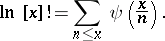How to Cite This Entry:
Chebyshev theorems on prime numbers. Encyclopedia of Mathematics. URL: http://encyclopediaofmath.org/index.php?title=Chebyshev_theorems_on_prime_numbers&oldid=16234
This article was adapted from an original article by A.F. Lavrik (originator), which appeared in Encyclopedia of Mathematics - ISBN 1402006098. See original article